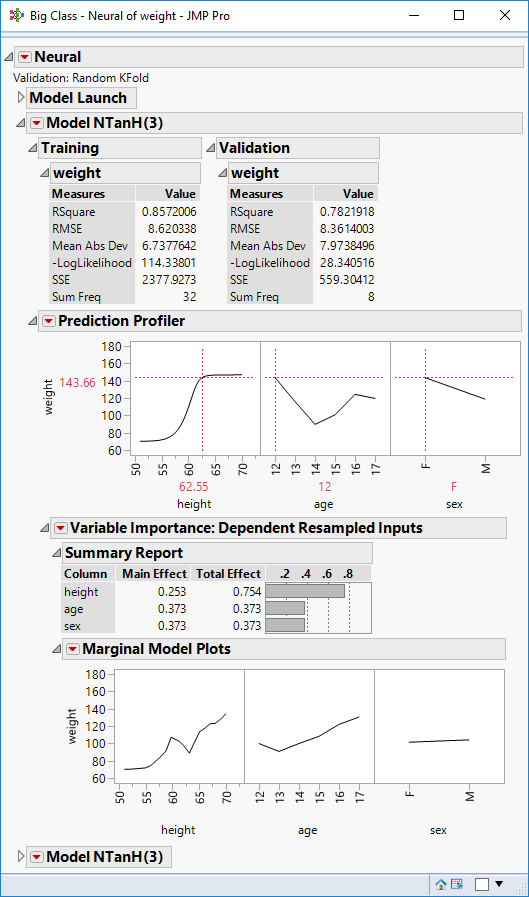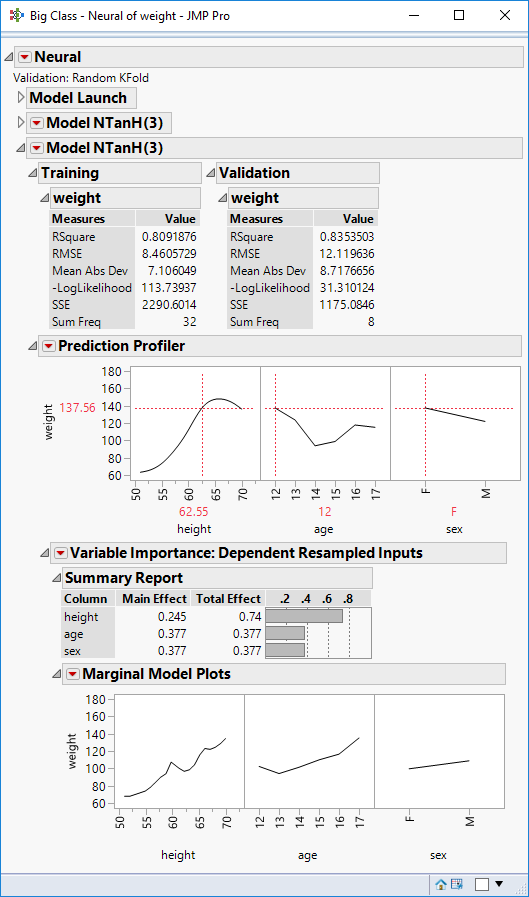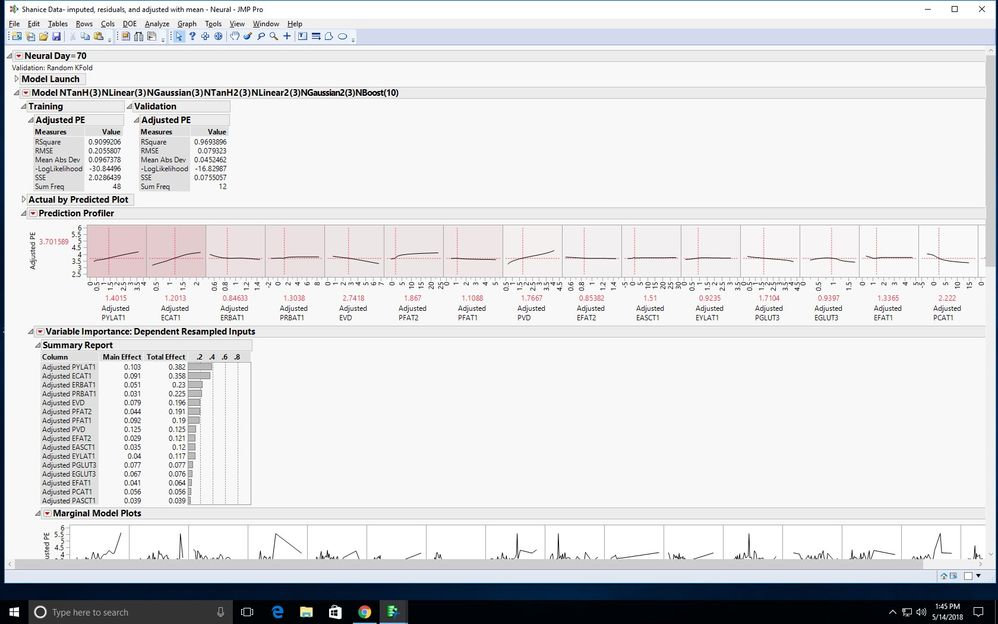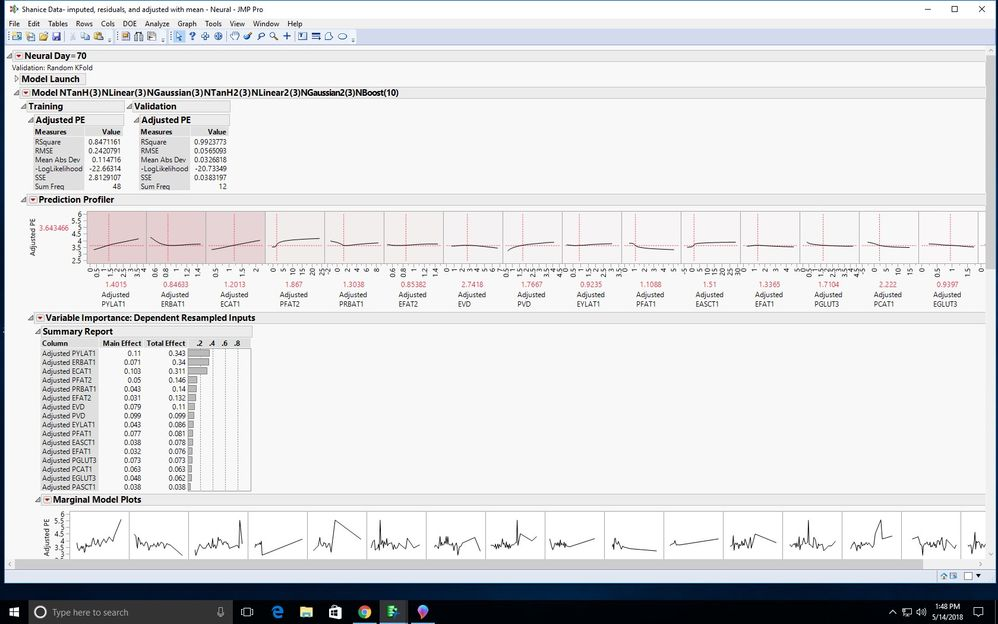Our World Statistics Day conversations have been a great reminder of how much statistics can inform our lives. Do you have an example of how statistics has made a difference in your life? Share your story with the Community!
Choose Language Hide Translation Bar
Highlighted

## Neural Networks

How reliable are neural networks? When I didn't set the seed I got different results so I set the seed, but when I change the seed from 1234 to 100 I also get different results. I'm interested in variable importance which completely changes each time I run the model. I've also tried increasing the number of tours but still variable importance changes. I'm using Kfold validation, 3 for hidden layer structure, 10 for number of models, 0.1 for learning rate, transform covariates and robust fit, squared penalty method and 20 number of tours. RSquare is around 0.9 for both training and validation.

Thank you,

Shanice Krombeen

7 REPLIES 7
Highlighted

## Re: Neural Networks

Neural networks are reliable predictive models. You are using k-fold cross-validation so, of course, each time you fit the model, you will get different estimates and results. The fact that you are getting practically the same R square for the training and validation sets is a good indication that the model is a good fit.

You can save the fitted model as a column formula. Then you don't have to fit the model again and the estimates won't change when you use the model.

Learn it once, use it forever!
Highlighted

## Re: Neural Networks

Should I simply choose the model with the highest R square? Each time I fit the model the R square is always high (between .90 and .94) but the variable importance under prediction profiler changes significantly.

Highlighted

## Re: Neural Networks

How many total observations do you have in your data set?

Learn it once, use it forever!
Highlighted

## Re: Neural Networks

There are 209 total observations in the data set but I'm fitting the model by day. Day 70 = 60, Day 90 = 91, and Day 110 = 58.

Highlighted

## Re: Neural Networks

So the sample size ranges from 60 to 91? That seems like sufficient data for k-fold CV. Still, if effects are small then the estimates and accompanying variable importance could change a lot with each random assignment to the training and validation sets. Here is a simple case based on the Big Class example and a NN using the default settings except validation uses 5-fold CV.(First fun)(Second run)

These two runs use the same data set and settings but involve different random assignments to the five folds. Is this variability similar to what you see?

Why not include Day as a predictor and then the data set size will be 209?

Learn it once, use it forever!
Highlighted

## Re: Neural Networks

Yes sample size ranges from 60 to 91. The variables are expression of different nutrient transporters in placenta or endometrium on day 70, 90, or 110 of gestation. Day is a significant effect and expression likely differs by day, which is why I fit the model by day. It is true that the effects are small so that may be why variable importance changes a lot. Here is an example of what I'm seeing.Neural Network Run 1Neural Network Run 2

Highlighted

## Re: Neural Networks

Fitting the NN by Day means that within each subset, each fold is 12-15 observations, so the stability of the estimates will be adversely affected.

That said, the top predictors are generally the same and they exhibit similar profiles in both runs. You can remove some of these predictors, perhaps as many as half, from the model with little loss in performance but the resulting parsimonious model will be more stable over NN runs.

Also, you are fitting a very complex model. There are 18 hidden nodes. Is the response really that complicated? Over-fitting the model will also affect the stability of the runs.

If the response is time-dependent as you say, then it makes more sense to me to include Day as a predictor and use the larger, combined data set for better stability.

Learn it once, use it forever!
Article Labels

There are no labels assigned to this post.